# Java写的斗地主游戏源码

+关注继续查看

这次采用的是JAVA8 ，最新发布的JAVA版本，

IDE是netbeans，一共有80MB左右大小，体积不大，安装也容易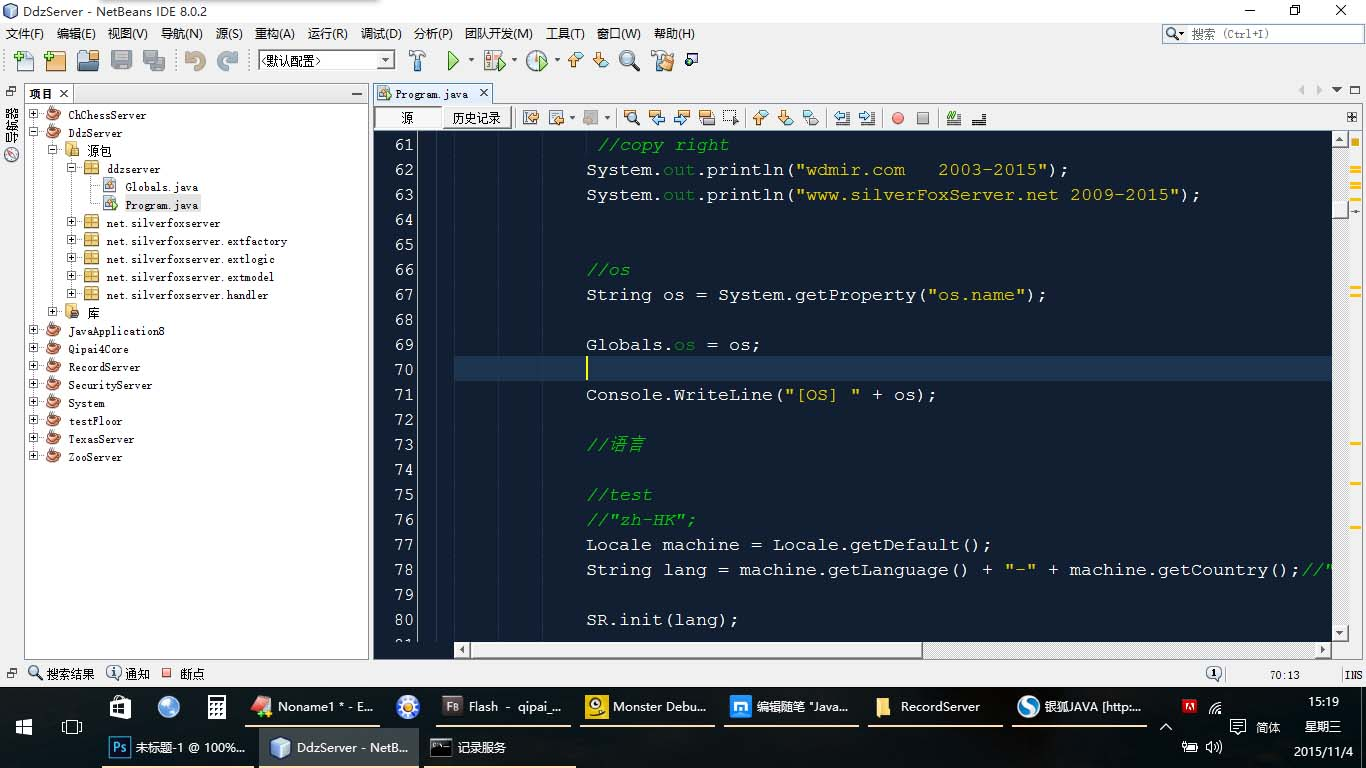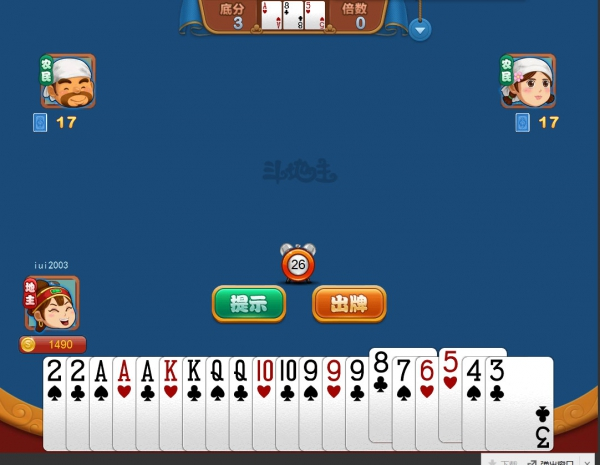《-                       《-             《-

现在开始构架游戏，为了不让代码那么难看，我们很有必要加入设计模式和面向对象思想。
首先，我们列出54张牌。

大家可以看到，扑克数字相同时，有4种花色，桃心梅方

利用这个特性，我们采用了数字间隔，0-3一组 ， 4-7一组，如果想得到花色，取模就可以了，是不是很方便？

 1 /**
2      * 背面牌都是负数
3      */
4     public static final int BG_NORMAL = -3;
5     public static final int BG_NONGMING = -2;
6     public static final int BG_DIZHU = -1;
7
8     public static final int F_3 = 0;
9     public static final int M_3 = 1;
10     public static final int X_3 = 2;
11     public static final int T_3 = 3;
12
13     public static final int F_4 = 4;
14     public static final int M_4 = 5;
15     public static final int X_4 = 6;
16     public static final int T_4 = 7;
17
18     public static final int F_5 = 8;
19     public static final int M_5 = 9;
20     public static final int X_5 = 10;
21     public static final int T_5 = 11;
22
23     public static final int F_6 = 12;
24     public static final int M_6 = 13;
25     public static final int X_6 = 14;
26     public static final int T_6 = 15;
27
28     public static final int F_7 = 16;
29     public static final int M_7 = 17;
30     public static final int X_7 = 18;
31     public static final int T_7 = 19;
32
33     public static final int F_8 = 20;
34     public static final int M_8 = 21;
35     public static final int X_8 = 22;
36     public static final int T_8 = 23;
37
38     public static final int F_9 = 24;
39     public static final int M_9 = 25;
40     public static final int X_9 = 26;
41     public static final int T_9 = 27;
42
43     public static final int F_10 = 28;
44     public static final int M_10 = 29;
45     public static final int X_10 = 30;
46     public static final int T_10 = 31;
47
48     public static final int F_J = 32;
49     public static final int M_J = 33;
50     public static final int X_J = 34;
51     public static final int T_J = 35;
52
53     public static final int F_Q = 36;
54     public static final int M_Q = 37;
55     public static final int X_Q = 38;
56     public static final int T_Q = 39;
57
58     public static final int F_K = 40;
59     public static final int M_K = 41;
60     public static final int X_K = 42;
61     public static final int T_K = 43;
62
63     public static final int F_A = 44;
64     public static final int M_A = 45;
65     public static final int X_A = 46;
66     public static final int T_A = 47;
67
68     public static final int F_2 = 56;
69     public static final int M_2 = 57;
70     public static final int X_2 = 58;
71     public static final int T_2 = 59;
72
73     public static final int JOKER_XIAO = 60;
74     public static final int JOKER_DA = 64;

/**
洗牌
*/
public final void xipai()
{
//
reset();

//
int i = 0;
int len = 0;
int n = 0;

//clone pai name
java.util.ArrayList<String> p = PAI_NAME.GetList();

//第一次发17张牌
len = 17;

//提高随机数不重复概率的种子生成方法:

//Millisecond 取值范围是 0 - 999
//DateTime.Now.Ticks是指从1970年1月1日（具体哪年忘了哈，好像是1970）开始到目前所经过的毫秒数——刻度数。

//54张牌的组合是 54!
//是一个非常大的数,结果是: 2.3e + 71
//因此我们的seed的取值范围也应该非常大,也就是0到上面的结果,
//Millisecond小了，导致只会出现999种牌的组合
//guid方法不可取,每回都是一样的

//直接以Random做为随机数生成器因为时钟精度问题，
//在一个小的时间段内会得到同样的伪随机数序列，
//你shuffle后会得到同一个结果。
//.net提供了RNGCryptoServiceProvider可以避免这种情况

//GetRandSeed后的取值范围是 0 - int32.MaxValue，虽然还差很远，但是999要好很多
java.util.Random r = new java.util.Random(RandomUtil.GetRandSeed());

for (i = 0; i < len; i++)
{
n = r.nextInt(p.size());

grid[i] = p.get(n);

p.remove(n);
}

for (i = 0; i < len; i++)
{
n = r.nextInt(p.size());

grid[i] = p.get(n);

p.remove(n);
}

for (i = 0; i < len; i++)
{
n = r.nextInt(p.size());

grid[i] = p.get(n);

p.remove(n);
} //end for

//底牌
grid2 = p.get(0);
grid2 = p.get(1);
grid2 = p.get(2);

//distory
p.clear();

}

过Win7的纸牌游戏的朋友，一定对于游戏中的发牌动画记忆深刻，现在我们自己来实现这个动画过程。提到发牌动画，90%的程序员肯定会想到利用位置（Location）的变化来刷新界面，可能需要启用一些线程或者计时器之类的。但是Flash天生就是用来做动画的，用Tween缓动可以很容易实现。

独立数据库，需要VPS或独立主机一台，有独立外网IP

1.将斗地主客户端所有文件 拷贝到论坛根目录

2.在服务器上安装好Java 8

修改DdzServer、RecordServer 目录里的run.bat，将里面路径修改为当前路

RecordServer 参数还包括连接论坛MYSQL数据库的用户名，密码等

依次启动DdzServer，RecordServer 和SecurityServer安全策略服务

Linux在terminal上输入 sudo java -jar 完整路径

*需要服务器开放9300 ，843 TCP端口

3.在浏览器输入 http://你的网址/ddz.php 开始游戏

https://github.com/wdmir/521266750_qq_com.gitJava模拟星空

12 0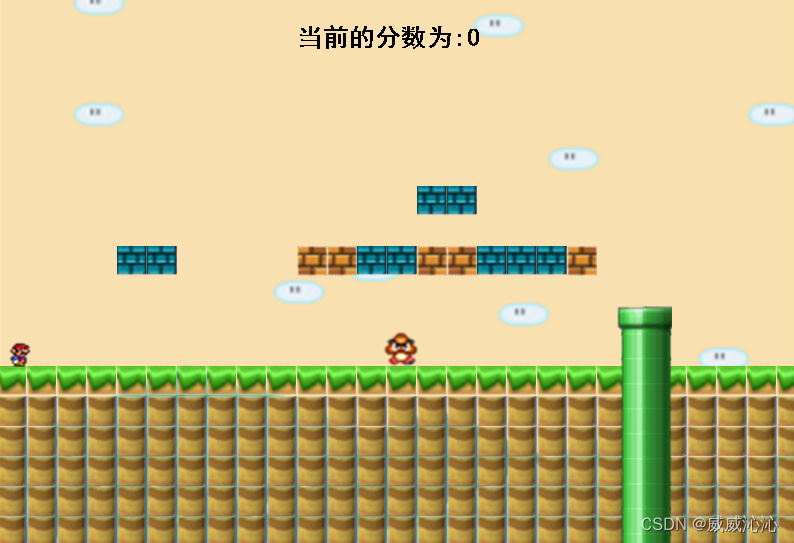java 实现超级玛丽小游戏
java 实现超级玛丽小游戏
64 016、Java——QuickHit游戏
16、Java——QuickHit游戏
64 0Java小游戏之飞机大战
java基础知识做的Java飞机大战小游戏，欢迎大家来看看。
93 0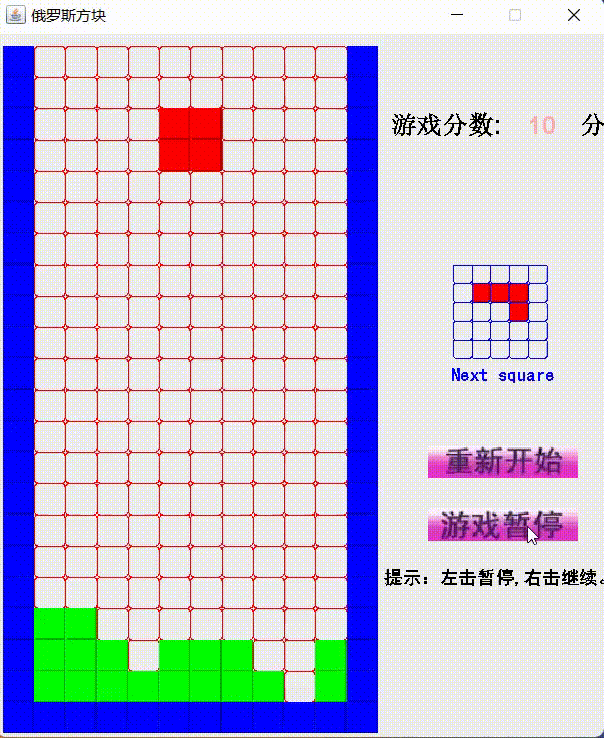99 0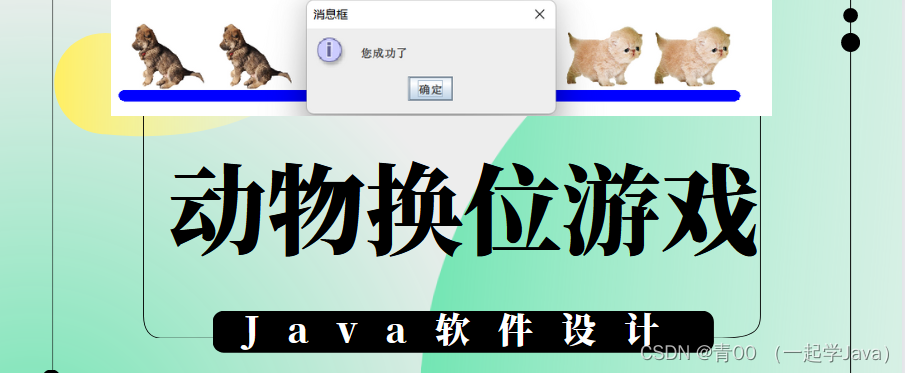java编程实战，基于Java的动物换位游戏【完整版】
197 0Java+Swing实现五子棋游戏（下)
Java+Swing实现五子棋游戏
74 0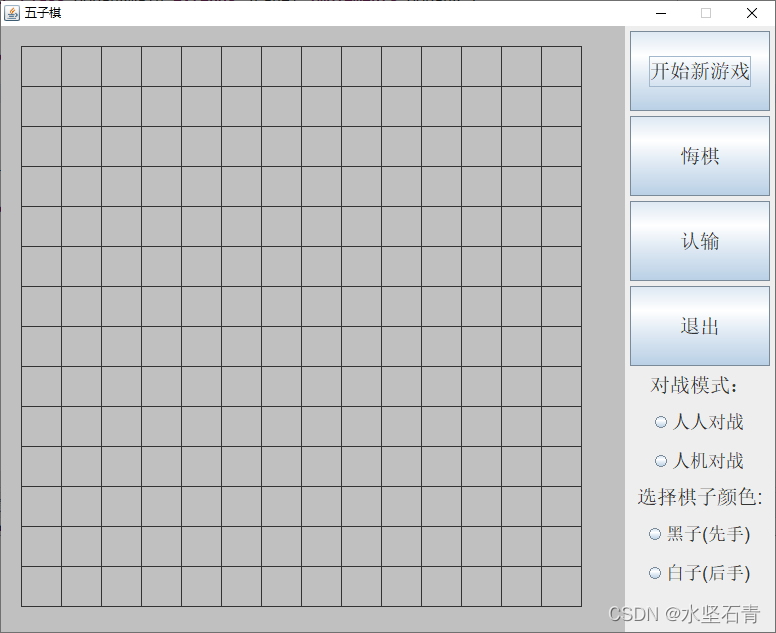Java+Swing实现五子棋游戏（上）
Java+Swing实现五子棋游戏
74 0

shop135452397.taobao.com

Java高级编程7780162

Java 开发手册（华山版）